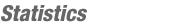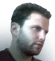JOINMatch Editorial
SRM 238
Thursday, April 14, 2005

Match summary

This SRM's early time slot seemed to enhance coder performance. In Division 2, the medium was submitted by more than 80% of the coders, and most were correct. The Div 1 Hard was correctly solved by 18 competitors. Kalmakka, with an amazingly fast submission on the hard problem, lead the pack going into the challenge round. The system tests claimed numerous solutions, and left misof on top by nearly 200 points. petr, competing for the second time, finished in second. Bhageera and pradhan_ptr took first and second place in Division 2.

# The Problems

ArrayHashUsed as: Division Two - Level One:
 Value 250 Submission Rate 291 / 307 (94.79%) Success Rate 285 / 291 (97.94%) High Score cypressx for 249.28 points (1 mins 32 secs) Average Score 228.79 (for 285 correct submissions)

To solve this problem, we iterate over the entire input and apply the given formula to each entry. An interesting feature of the formula, is that the position of each letter has no effect on the final return value. The important factors are the number of times each letter occurs, the number of elements in the input, and the number of characters in each element of the input. Java code follows :

```public int getHash(String[] input) {
int ret = 0;
for (int i = 0; i < input.length; i++)
for (int j = 0; j < input[i].length(); j++)
ret += input[i].charAt(j) - 'A' + i + j;
return ret;
}
```

PrintSchedulerUsed as: Division Two - Level Two:
 Value 500 Submission Rate 252 / 307 (82.08%) Success Rate 224 / 252 (88.89%) High Score Voldemort for 487.32 points (4 mins 36 secs) Average Score 379.96 (for 224 correct submissions)
Used as: Division One - Level One:
 Value 200 Submission Rate 201 / 201 (100.00%) Success Rate 198 / 201 (98.51%) High Score Eryx for 198.94 points (2 mins 4 secs) Average Score 183.46 (for 198 correct submissions)

In this problem we must write code that simulates a multithreaded system. Since we are explicitly given the time slices alocated to each thread, the solution amounts to simple iteration code. In a separate array, we maintain the position of each thread, so that subsequent executions begin properly. Java code follows:

```public String getOutput(String[] threads, String[] slices) {
StringBuffer ret = new StringBuffer();
for (int i = 0; i < slices.length; i++) {
String[] toks = slices[i].split(" ");
int threadNum = Integer.parseInt(toks), time = Integer.parseInt(toks);
for (int j = 0; j < time; j++) {
}
}
return ret+"";
}
```

RedundantStringsUsed as: Division Two - Level Three:
 Value 1000 Submission Rate 64 / 303 (21.12%) Success Rate 50 / 64 (78.12%) High Score CatalinT for 859.01 points (11 mins 55 secs) Average Score 576.76 (for 50 correct submissions)

The key to this problem is given in the statement. Every redundant string has a unique non-redundant root. To count the number of redundant strings of a given length, we only need to consider the possible roots. Based on a divisibility argument, the length of a root must divide the length of the string. Thus, to find all non-redundant roots, we iterate over all possible divisors. Java code follows:

```public int howMany(int length) {
if (length == 1) return 0;
int ret = 0;
for (int sub = 1; sub < length; sub++)
if (length % sub == 0)
//# of Non-Redundant = Total - Redundant
ret += (1 << sub) - howMany(sub);
return ret;
}```

SequenceSyncUsed as: Division One - Level Two:
 Value 500 Submission Rate 113 / 201 (56.22%) Success Rate 58 / 113 (51.33%) High Score misof for 481.52 points (5 mins 36 secs) Average Score 342.11 (for 58 correct submissions)

To solve this problem, we perform a breadth first search over all possible machine configurations. Each configuration is a set containing the states that the machine could be in. Initially, the machine could be in any state, so the set of all states is given a cost of 0. Considering all possible input symbols, we can compute which sets are within 1 step of the initial set. This process is continued until we find a set containing a single element. If no such singleton set is reachable, our method returns -1.

A similar way of looking at this problem involves non-determinism. Assume the given machine is an NFA. Perform the full subset construction to get a DFA. Make the set of all states the start state, and all singleton states final. The problem asks for the length of the shortest accepted string. Although this method gives a loose exponential upper bound, a tighter cubic bound is not hard to prove. It has been conjectured, that the maximum possible answer for an n state input is (n-1)2.

SquareLanguageUsed as: Division One - Level Three:
 Value 1000 Submission Rate 32 / 201 (15.92%) Success Rate 18 / 32 (56.25%) High Score kalmakka for 885.33 points (10 mins 30 secs) Average Score 579.81 (for 18 correct submissions)

This is one of those problems that becomes extremely easy to code once you have the right idea. Each string in S2 will look as follows:

```       #   #   #   #   #   #   #   #
--- --- --- --- --- --- --- ---
a   b   c   d   a   b   c   d
```
Each of the #s correspond to some quantity of the letter below it. If all of the lower bounds are positive, we can easily compute the solution by multiplying the number of possible values for each #. In fact, this condition can be weakened: if at least 2 of the lower bounds are positive, the solution can be computed via simple multiplication (why?). When this condition is not satisfied, the simple method may overcount certain strings. The case where fewer than 2 lower bounds are positive will be handled separately.

Suppose, without loss of generality, the b's have a positive lower bound, but all other letters have 0 as a lower bound. The simple multiplication method will overcount the case where the c's, d's, and a's between the two groups of b's are all missing. For example, the case where the first group has 5 b's and the second group has 7 will be considered different from the case where the first group has 10 b's and the second group has 2. The following formula describes how far the simple method overcounts:
```      (B - 1)2*A*C*D
```
Here A, B, C, and D denote the number of possible values for a's, b's, c's and d's. For example, C = uc - lc + 1. Note that
```   (B - 1)2 = B2 - (2B - 1)
```
When the letters between the b's are missing, the simple method counts B*B*A*C*D strings, but in reality there are only (2B-1)*A*C*D strings. When all 4 letters have lower bounds at 0, this formula must be subtracted 4 times, for each potential case of overcounting. Java code follows:
```public long howMany(int[] abounds, int[] bbounds, int[] cbounds, int[] dbounds) {
int[][] arrs = {abounds,bbounds,cbounds,dbounds};
int cnts[] = new int, lowSum = 0;
long ret = 1, simp = 0;
for (int i = 0; i < cnts.length; i++) {
simp = ret *= cnts[i] = arrs[i] - arrs[i] + 1;
lowSum += arrs[i];
}
ret *= ret;
for (int i = 0; i < 4; i++)
if (arrs[i] == lowSum)
ret -= simp*(cnts[i] - 1)*(cnts[i] - 1)/cnts[i];
return ret;
}
```By brett1479
TopCoder Member